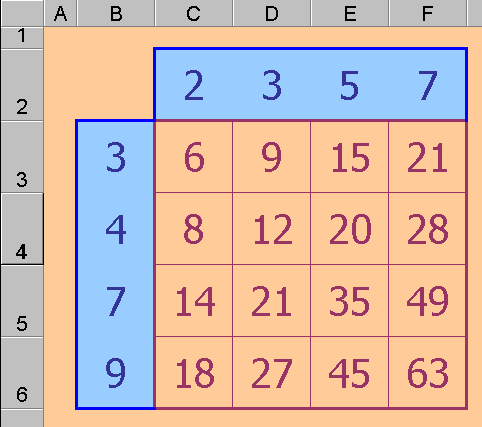# Excel Technique: Making a Table for a Function of Two Independent

##### Age 11 to 16Challenge Level
As an example here is a simple Tables grid:The cell C3 contains the formula: = C\$2 * \$B3

* means multiply in Excel, and although the functions we need to investigate will often involve more than simple multiplication, the process explained below is easily applied even when the function itself is complicated.
In the illustration above, C2 is the factor from the top row and B3 is the factor from the left hand column. The dollar sign, \$, used in the C3 formula, ensures that when the formula is copied from C3 across the whole C3:F6 range the first factor will continue to be taken from row 1 and the second factor will still be taken from column A. Once the correct formula (above) has been entered in C3, the keystrokes for the copy manoeuvre are: • select C3 (just click on it) • choose Copy from the Edit menu • highlight (click and drag) the C3:F6 range • choose Paste from the Edit menu. The formula from C3 is copied automatically to all cells in the C3:F6 range. The formula is adjusted for each new position except where a \$ sign was placed to show that this automatic assistance is unwanted.